21.  Which has maximum value of mean free path ?

A. $$CO_{2}$$

B. $$H_{2}$$

C. $$O_{2}$$

D. $$N_{2}$$

22.  4.0 gm ideal gas is filled in a bulb having volume 10 $$dm^{3}$$ at a constant temperature T & constant pressure P. If 0.8 gm gas is removed from the bulb to maintain the original pressure at (T + 125)K temperature, what would be the value of T for a gas having molar mass 40 $$gm\ mole^{-1}$$.

A. 500K

B. $$500^{\circ}C$$

C. 773K

D. $$773^{\circ}C$$

Explanation :

Pressure and Temperature are constant

So $$n_{1}T_{1}=n_{2}T_{2}$$

$$\frac{W_{1}}{M_{1}}\times T_{1}=\frac{W_{2}}{M_{2}}\times T_{2},\ M_{1}=M_{2}$$

$$W_{1}T_{1}=3.2(T+125)$$

$$4T=3.2T+400$$

$$\therefore 0.8T=400$$

$$\therefore T=500K$$

23.  What would be value of ratio for RMS and average speed of gaseous molecules at a constant temperature ?

A. 1.086 : 1

B. 1 : 1.086

C. 2 : 1.086

D. 1.086 : 2

24.

If temperature $$T_{2} > T_{1}$$, which graph of maxwell Botlzmamn distriburibution of molecular speed iscorrect ?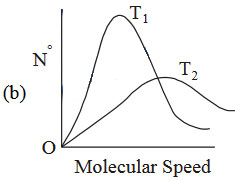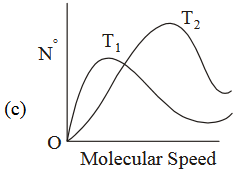A. a

B. b

C. c

D. d

25.  The RMS velocity of an ideal gas at constant pressure varies with density relates as

A. $$d$$

B. $$\sqrt{d}$$

C. $$d^{2}$$

D. $$\frac{1}{\sqrt{d}}$$

26.  The ratio of rms (root mean square ) velocities for two different gases is

A. $$\frac{\overline{V_{1}}}{\overline{V_{2}}}=\sqrt{\frac{M_{2}}{M_{1}}}$$

B. $$\frac{m_{1}}{\sqrt{M_{2}}}=\frac{m_{2}}{\sqrt{M_{1}}}$$

C. $$\frac{\alpha _{1}}{\sqrt{M_{2}}}=\frac{\alpha _{2}}{\sqrt{M_{1}}}$$

D. $$\frac{\sqrt{M_{2}}}{m_{1}}=\frac{\sqrt{M_{1}}}{m_{2}}$$

27.  At constant temperature, a gas is filled at 1 atm pressure in a closed container. To compress this gas to $$\left (\frac{1}{4} \right )^{th}$$ of its initial volume, the pressure to be applied is

A. $$\frac{4}{3}\ atm$$

B. $$2\ atm$$

C. $$\frac{1}{4}\ atm$$

D. $$\frac{1}{3}\ atm$$

28.  What is the pressure of 380 mm Hg column of a gas in pascal ?

A. $$5.05\times 10^{4}\ Pa$$

B. $$5.06\times 10^{5}\ Pa$$

C. $$0.505\times 10^{3}\ Pa$$

D. $$1.013\times 10^{5}\ Pa$$

Explanation :

$$380\ mm=380\ torr=\frac{380}{760}\ atm$$

$$\therefore 0.987\ atm=10^{5}\ Pa$$

$$\therefore \frac{380}{760}\ atm=?$$

$$\therefore 380\ mm=5.05\times 10^{4}\ Pa$$

29.

The graphs plotted $$V\rightarrow T$$ for one mole of an ideal gas as follows , which graph represent its ideal behaviour at atmospheric pressure ?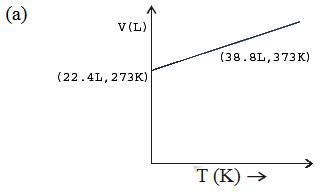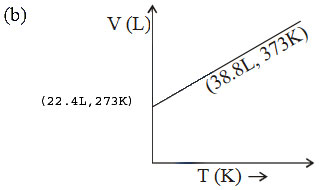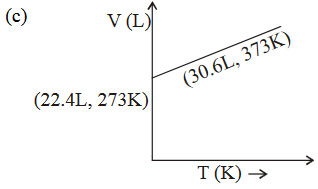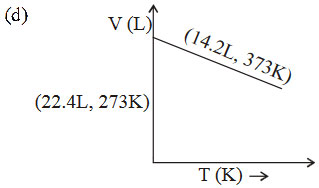A. a

B. b

C. c

D. d

Explanation :

At const pressure according to charles law$$\frac{V_{1}}{T_{1}}=\frac{V_{2}}{T_{2}}=K$$

$$\therefore \frac{22.4}{273}=0.082$$ .......... (I)

and $$\therefore \frac{30.6}{373}=0.082$$ ..........(II)

(I) and (II) constant behaves the gas as an ideal

30.  Helium gas is compressed to half of the volume at 303 K. It should be heated to which temperature for its volume to increase to double of its original volume ?

A. 303K

B. 606K

C. 1212K

D. $$30^{\circ}C$$

Explanation :

According to Charles’ law

$$\frac{V_{1}}{T_{1}}=\frac{V_{2}}{T_{2}}=K$$  $$V_{1}=\frac{V_{1}}{2}$$ at $$T_{1}=303K$$

$$\therefore T_{2}=\frac{V_{2}T_{1}}{V_{1}}$$   $$V_{2}=2V_{1}$$ at $$T_{2}=?$$

$$=\frac{2V_{1}\times 303}{\frac{V_{1}}{2}}$$

= 303 x 4

= 1212K